## Distance for Binary Variables

We often face variables that only binary value such as Yes and No, or Agree and Disagree, True and False, Success and Failure, 0 and 1, Absence or Present, Positive and Negative, etc. For such binary variables, there are only two possible values, which can be represented as positive and negative. Similarity of dissimilarity (distance) of two objects that represented by binary variables can be measured in term of number of occurrence (frequency) of positive and negative in each object.

For example:

 Feature of Fruit Sphere shape Sweet Sour Crunchy Object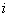=Apple Yes Yes Yes Yes Object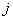=Banana No Yes No No

The coordinate of Apple is (1,1,1,1) and coordinate of Banana is (0,1,0,0). Because each object is represented by 4 variables, we say that these objects has 4 dimensions.

Let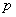= number of variables that positive for both objects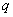= number of variables that positive for theth objects and negative for theth object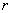= number of variables that negative for theth objects and positive for theth object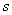= number of variables that negative for both objects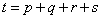= total number of variables

 ObjectYes No objectYesNoFor our example above, we have measured Apple and Banana have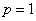,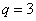and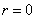,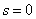. Thus,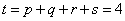The most common use of binary dissimilarity (distance) is

B.S. Everit (1978) listed 10 other similarity measures for presence-absence data that have been proposed

1.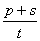= simple matching coefficient
2.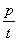3.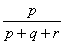= Jaccard's coefficient
4.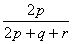5.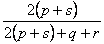6.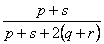7.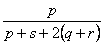8.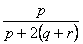9.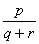10.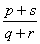This tutorial is copyrighted.

Preferable reference for this tutorial is

Teknomo, Kardi (2015) Similarity Measurement. http:\people.revoledu.comkardi tutorialSimilarity